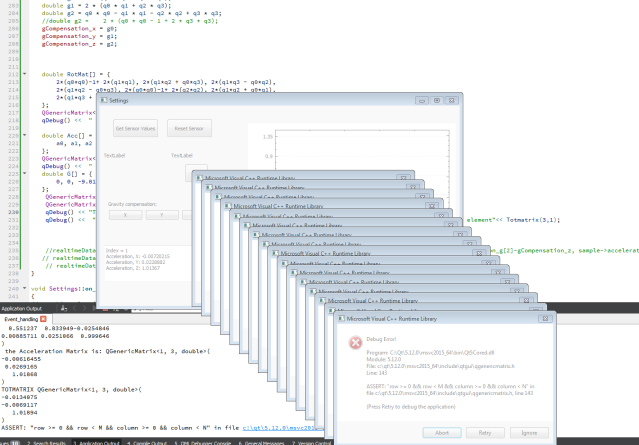# Error indexing a QGenericMatrix

• Hi All,

I do the following matrix manipulation:

`````` double RotMat[] = {
2*(q0*q0)-1+ 2*(q1*q1), 2*(q1*q2 + q0*q3), 2*(q1*q3 - q0*q2),
2*(q1*q2 - q0*q3), 2*(q0*q0)-1+ 2*(q2*q2), 2*(q1*q2 + q0*q1),
2*(q1*q3 + q0*q2), 2*(q2*q3 - q0*q1), 2*(q0*q0)-1+ 2*(q3*q3)
};
QGenericMatrix<3,3,double> rotationmatrix(RotMat);

double Acc[] = {
a0, a1, a2
};
QGenericMatrix<1,3,double> accelmatrix(Acc);

QGenericMatrix<1,3,double> Totmatrix = rotationmatrix*accelmatrix;
``````

where q0,q1,q2,q3 and a0,a1,a3 are realtime values from a sensor

Then if I print `TotMatrix` my system is getting crashed! (screenshot)Any idea what might be the cause for this?

Thank you

• Any idea what might be the cause for this?

As always when you encounter a crash: Use a debugger and take a look at the backtrace to see where exactly it happens and why.

• I feel your `accelmatrix` is a row vector, which cannot be multiplied by a rotation matrix in that order. Either use a column vector or write `accelmatrix * rotationmatrix` (which would use the inverse rotation).

• @Christian-Ehrlicher @JohanSolo Thanks for your feedback.

I feel like it's a problem of indexing.

``````   double a[] = {
0.5,0.8, 0.7
};
QGenericMatrix<3,1,double> A(a);
``````

What's the correct way to index each of `A` matrix's element?
I get runtime error If I do this:

`````` qDebug() <<  "Element at 1,1:"<< A(1,1);
``````

It is pointing at `qgenericmatrix.h`

``````template <int N, int M, typename T>
Q_INLINE_TEMPLATE T& QGenericMatrix<N, M, T>::operator()(int row, int column)
{
Q_ASSERT(row >= 0 && row < M && column >= 0 && column < N); //------> error pointing here
return m[column][row];
}
``````

What I'm doing wrong here?

• In C, C++ and other useful "real" languages, arrays and matrices are zero-indexed.

• @JohanSolo Thanks a lot :)

• double RotMat[] = {
2*(q0q0)-1+ 2(q1q1), 2(q1q2 + q0q3), 2*(q1q3 - q0q2),
2*(q1q2 - q0q3), 2*(q0q0)-1+ 2(q2q2), 2(q1q2 + q0q1),
2*(q1q3 + q0q2), 2*(q2q3 - q0q1), 2*(q0q0)-1+ 2(q3*q3)
};

This looks like quaternion multiplication :)
You might want to have a look at `QQuaternion` which you can convert to `QMatrix` and `QVector3D`
https://doc.qt.io/qt-5/qquaternion.html

• @Pl45m4 Thanks a lot for your suggestion :)

I can't find an example of using `QQuaternion` class. I have a question, hope you can give me some feedback.
I have quaternion coming from the IMU in the form of `w`,`x`, `y`,`z`. (`w` is the scalar and others are vectors). Also acceleration vectors `ax, ay, az`. I need to rotate the accelerometer reading by the quaternion into the Earth frame of reference. How can I make use of `QQuaternion` class to perform this?

• @viniltc

This will rotate your vector or given axis by a given quaternion.
It's the same as using the rotation operator `qvq*`

``````QQuaternion q; // your Q
QVector3D vector(ax,ay,az);

QVector3D result = q.rotatedVector(vector);
``````

https://doc.qt.io/qt-5/qquaternion.html#rotatedVector

• @Pl45m4 Thanks a lot :)

• @Pl45m4
I need to multiply a vector by the inverse quaternion. The Idea is to compensate gravity from acceleration vector. I did the following steps:

Step 1. Quaternion multiplication (`v' = qvq*`)
I did this:

``````QQuaternion q;
QVector3D aVector(ax,ay,az);
QVector3D qRotated = q.rotatedVector(vector); // this is v'
``````

Step 2. Substarct gravity vector from the above rotated vector (`v'' = qvq*-g`) , `g` is gravity vector:

``````QVector3D g(0,0,1);
QVector3D result = qRotated-g; // this is v''
``````

Step 3. I need to multiply the `result` by inverse quaternion (`v''' = q-1 . v'' . q-1*`)

I tried this (but I'm not sure if it's the right way to do it!):

``````QQuaternion quatinv= quat.inverted();
QVector3D noGRotated= quatinv.rotatedVector(result);
``````

the `noGRotated` (or `v'''`) should be the original acceleration measurement minus gravity. But there is something wrong with the calculation it seems, ideally if I take measurements while the device is stationary. No matter how the device is rotated, the acceleration measurements should be close to (0,0,0), but it is not in my case.

Do you see any issues in the Step 2 and 3 above?

• @viniltc

Why you need the inverse of the resulting quaternion in step 3?
I've never done gravity compensation before, but I think it may works like suggested here:

There are also some papers dealing with this topic.

I would try to just subtract the gravity component (vector in Z - direction, let's say `(0, 0, 9.81)`) from your actual, accelerometer vector.

Is the result vector after step 2 looking correct?